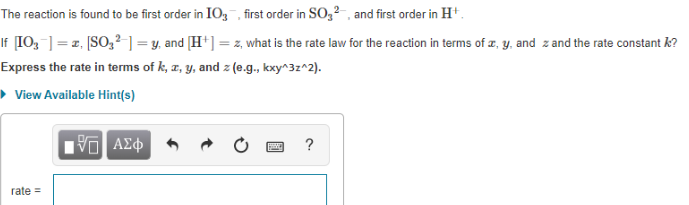# The reaction is found to be first order in IO3^-, first order in SO3^2-, and first order in H+. If [IO3^-] = x, [SO3^2-] = y, and [H^+] = z, what is the rate law for the reaction in terms of x, y, and z and the rate constant k? Express the rate in terms of k, x, y, and z(e.g., kxy^3z^2).